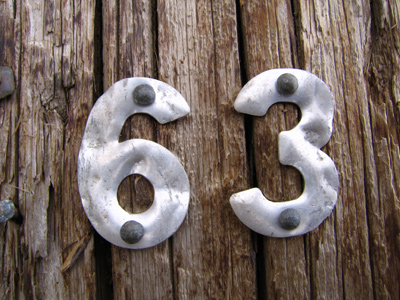13 + 14 + 36 = 63.

Subtraction is taking away a number from another number. Addition and subtraction are important skills: you've got to be able to check that you've been given the right change, haven't you?

This 11-plus Maths quiz will give you a bit of practice in adding and subtracting.

TIP: With numbers less than 100, add the tens first and then add the ones. For example:

• 12 + 37 + 47 = (10 + 30 + 40) + (2 + 7 + 7) = 80 + 16 = 96

Try to do this stuff in your head - that way next time you are out shopping, you'll know immediately if you've been short-changed or not!

In each of the following questions, what is the answer to the given problem?
1.
47 - 58 + 19 = ?
8
7
6
9
TIP: With numbers less than 100, add the tens first and then add the ones. 47 - 58 + 19 = (40 + 20 - 50) + (7 - 8 - 1) = 10 - 2 = 8. Here '19' was replaced by '20 - 1' and the calculation was done using this
2.
3 - 6 + 17 + 28 = ?
44
42
43
46
TIP: With numbers less than 100, add the tens first and then add the ones. 3 - 6 + 17 + 28 = (10 + 20) + (7 + 8 + 3) - (6) = 30 + 18 - 6 = 48 - 6 = 42
3.
19 - 27 + 56 = ?
49
51
48
53
TIP: With numbers less than 100, add the tens first and then add the ones. 19 - 27 + 56 = (10 + 50 - 20) + (9 + 6 - 7) = 40 + 8 = 48. You could write '19' as '20 - 1' and do the calculation using this. Always BE ON THE LOOKOUT for useful simplifications
4.
13 + 14 + 36 = ?
63
61
62
65
TIP: With numbers less than 100, add the tens first and then add the ones. 13 + 14 + 36 = (10 + 10 + 30) + (3 + 4 + 6) = 50 + 13 = 63. Don't forget: sometimes you will spot an easy addition which will make the calculation easier, e.g. 14 + 36 = 50
5.
27 + 46 + 83 = ?
168
158
165
156
TIP: With numbers less than 100, add the tens first and then add the ones. 27 + 46 + 83 = (20 + 40 + 80) + (7 + 6 + 3) = 140 + 16 = 156
6.
37 - 19 = ?
19
16
18
17
TIP: With numbers less than 100, add the tens first and then add the ones. 37 - 19 = (30 - 10) + (7 - 9) = 20 - 2 = 18
7.
25 - 47 + 89 - 5 = ?
61
62
64
63
TIP: With numbers less than 100, add the tens first and then add the ones. 25 - 47 + 89 - 5 = (20 + 80 - 40) + (5 + 9 - 7 - 5) = 60 + 2 = 62
8.
6 + 6 + 6 - 6 = ?
18
24
12
6
TIP: With numbers less than 100, add the tens first and then add the ones. (6 + 6 + 6) - 6 = 18 - 6 = 12
9.
0 + 10 + 0 + 28 = ?
48
58
0
38
TIP: With numbers less than 100, add the tens first and then add the ones. 0 + 10 + 0 + 28 = (10 + 20) + (8) = 30 + 8 = 38. Ignore zeros!
10.
16 + 17 = ?
35
36
33
37
TIP: With numbers less than 100, add the tens first and then add the ones. 16 + 17 = (10 + 10) + (6 + 7) = 20 + 13 = 33
Author:  Frank Evans